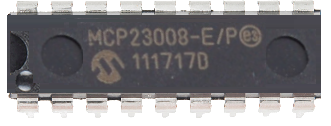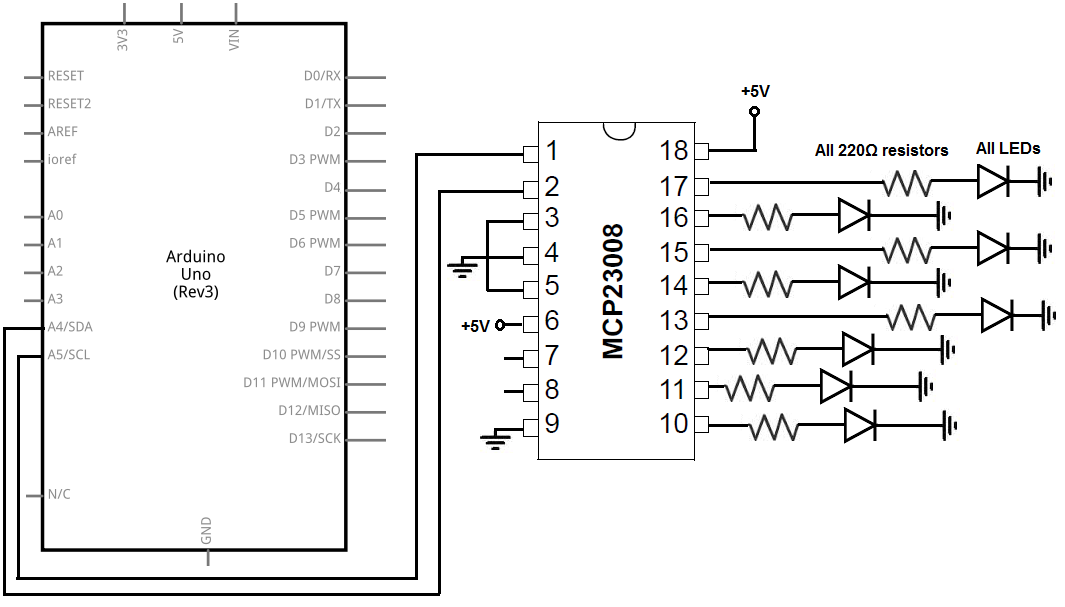﻿ How to Connect an MCP23008 I/O Port Expander to an Arduino ﻿# How to Connect an MCP23008 I/O Port Expander to an ArduinoIn this project, we will show how to connect an MCP23008 I/O port expander chip to an Arduino microcontroller.

This is a very useful thing to do if we need additional I/O ports for a microcontroller.

I/O ports are input/output ports.

If we need more inputs or outputs to a microcontroller, we can expand the number of I/O ports with an I/O port expander chip.

The MCP23008 chip will let us have an additional 8 I/O ports simply by just using 2 pins on the microcontroller.

The MCP23008 chip uses the I2C bus and protocol. So only 2 digital pins on the arduino need to be used. So it uses a very minimal number of I/O pins for communication.

The 2 pins that it uses are for the clock line and data line.

Each of the I/O ports are bidirectional. So the device attached to one of the ports can both receive and send information to the microcontroller. Each of the ports can sink up to 25mA of current, so this is enough to drive certain devices like LEDs, which we use in this circuit. If you need more current you can always use a transistor to get more, but for this circuit, the IC is capable of driving LEDs.

Being that an MCP23008 contains 8 outputs, we can drive 8 LEDs with it.

Components

• MCP23008 I/O Port Expander
• A few 220Ω resistors
• A few LEDs
• Arduino microcontroller

The MCP23008 I/O port expander can be obtained for a little over \$2.

It is a perfect addition to a microcontroller where more I/O ports are needed.

It is a 18-pin chip.

The datasheet for this chip can be found at the following link: MCP23008 Datasheet.

The pinout of the MCP23008 is shown below.The MCP23008 can be powered with +5V. Therefore, we can connect VDD to the 5V terminal of the arduino. We connect VSS to ground. This completes the powering that's necessary for the MCP23008.

The GP0-GP7 are the 8 I/O ports.

NC is Not Connected so we leave that pin unconnected (or floating).

SCL is the serial clock line. It connects to analog pin 5 on the arduino, which is the analog clock pin.

SDA is the serial data line. It connects to analog pin 4 on the arduino, which is the analog data line.

INT is the interrupt pin for the outputs. Being that we are not going to use interrupts here, we just leave this pin unconnected.

The RESET pin is if you want the outputs all reset to 0. Being that we aren't going to use this pin, we connect it permanently to +5V.

A0, A1, and A2 are the address pins. You only need be concerned about these pins if you are using multiple MCP23008 chips. These pins are externally biased, meaning you provide voltages to them to create different addresses. Since there are 3 pins, you can create a total of 8 different addresses (23=8). This is only necessary if you are connecting multiple ICs, so that we can distinguish between them. For example, if I grounded A2 and A1 and held A0 HIGH, this would create the address 001. Being that we are grounding all 3 pins, the address will for the address pins will be 000. But if you are connecting up to 8 MCP23008s, you will need to configure these pins so that each has a unique address: 000, 001, 010, 011, 100, 101, 110, 111. This way, you will be able to uniquely address each of them.

### MCP23008 I/O Port Expander Circuit with an Arduino Microcontroller

The MCP23008 I/O port expander circuit we will build with an Arduino microcontroller is shown below.In this circuit, the hardware connections are very simple.

To each of the I/O pins, we attach a 220Ω resistor and an LED.

To power the pin, we attach +5V to VDD and connect VSS to GND. This establishes power to the MCP23008 chip.

We leave the NC (Not Connect) pin unconnected (or floating).

We connect pin 1 of the MCP23008, which is SCL (serial clock line) to analog pin 5 of the arduino. This allows for clock synchrony between the arduino and the I/O port expander chip.

We connect pin 2 of the MCP23008, which is the SDA (serial data line) to analog pin 4 of the arduino. This allows data transfer between the arduino and the I/O port expander chip.

Being that we're not working with interrupts, we leave INT pin unconnected.

Being that we don't want to use the RESET pin, we connect it to +5V, since it's active LOW. However, if you want to use this pin, you simply connect it to a digital pin on the arduino. If you want to reset all the outputs, then you draw this pin LOW in code.

And we connect the address pins, pins A0, A1, and A2, to ground. This makes the address of these 3 pins 000. Later on in our code, this will be important for addressing this MCP23008 chip.

### Code

The code so that we can connect an MCP23008 I/O port expander to an arduino microcontroller to turn on LEDs is shown below.

Arduino's I2C communication library is called the Wire library. With this library, you can easily write to and read from I2C devices.

#include <Wire.h>

void setup()
{
Wire.begin(); //creates a Wire object

// set I/O pins to outputs
Wire.beginTransmission(0x20); //begins talking to the slave device
Wire.write(0x00); //selects the IODIRA register
Wire.write(0x00); //this sets all port A pins to outputs
Wire.endTransmission(); //stops talking to device
}

void loop()
{
Wire.beginTransmission(0x20); //starts talking to slave device
Wire.write(0x09); //selects the GPIO pins
Wire.write(00000011); // turns on pins 0 and 1 of GPIOA
Wire.endTransmission(); //ends communication with the device
}

First, we import the Wire library, which is the library for communicating with I2C devices.

Then we select the IODIR register. The address for this register is 0x00. This can be found in the datasheet. All the addresses of registers that we need for programming can be found in the MCP23008 datasheet. The IODIR is the register of all the 8 pins in port A. The next line then sets all the pins to 0, which makes them outputs. If we wanted to set all the pins to inputs, we would set the value to all 1s. In hexadecimal, this would be 0xff. We can also make some pins inputs and some outputs with the appropriate value.

Next is our loop function which repeats over and over again. Here we will write which pins are on and which are off.

First, we begin communication with the MCP23008 chip, which always must be done. Then we write the line, Wire.write(0x09). This selects the GPIO pins, which are the I/O pins. The difference between IODIR and GPIO is that IODIR only can control whether a pin is input or output. GPIO doesn't control that but now controls whether that pin turns on or off. Writing a 1 to the pin turns it on, while writing a 0 turns it off. Here, we select the GPIO register and then write the value in binary so you can see the exact bits which are on and off. Here, we turn on pins 0 and 2 and leave all the other pins off.

There are several variations you can do to this circuit. If you want to blink LEDs, then you would turn the LED on, delay for a certain time period, and then turn it off. For example, if you wanted to blink an LED on pin 0, you would write, Wire.write(00000001), then maybe wait a half of second, so you would write, delay(500), then you would turn off the LED by the line, Wire.write(00000000). So there are so many variations that can be done with this circuit. And it can really function just like a regular microcontroller, just with extended I/O port capability.

If you want to turn all the pins on, then you either write, Wire.write(00000011) or Wire.write(0xff). Both turn on the LEDs on.

You just have to get used to the physical wiring and code involved with extending the I/O ports on a given microcontroller. Once you do this and get the hang of it, then it's pretty straightforward.

Related Resources

How to Connect an MCP23017 I/O Port Expander to an Arduino Microcontroller